# 4.2 Graphs of exponential functions  (Page 6/6)

 Page 6 / 6

## Verbal

What role does the horizontal asymptote of an exponential function play in telling us about the end behavior of the graph?

An asymptote is a line that the graph of a function approaches, as $\text{\hspace{0.17em}}x\text{\hspace{0.17em}}$ either increases or decreases without bound. The horizontal asymptote of an exponential function tells us the limit of the function’s values as the independent variable gets either extremely large or extremely small.

What is the advantage of knowing how to recognize transformations of the graph of a parent function algebraically?

## Algebraic

The graph of $\text{\hspace{0.17em}}f\left(x\right)={3}^{x}\text{\hspace{0.17em}}$ is reflected about the y -axis and stretched vertically by a factor of $\text{\hspace{0.17em}}4.\text{\hspace{0.17em}}$ What is the equation of the new function, $\text{\hspace{0.17em}}g\left(x\right)?\text{\hspace{0.17em}}$ State its y -intercept, domain, and range.

$g\left(x\right)=4{\left(3\right)}^{-x};\text{\hspace{0.17em}}$ y -intercept: $\text{\hspace{0.17em}}\left(0,4\right);\text{\hspace{0.17em}}$ Domain: all real numbers; Range: all real numbers greater than $\text{\hspace{0.17em}}0.$

The graph of $\text{\hspace{0.17em}}f\left(x\right)={\left(\frac{1}{2}\right)}^{-x}\text{\hspace{0.17em}}$ is reflected about the y -axis and compressed vertically by a factor of $\text{\hspace{0.17em}}\frac{1}{5}.\text{\hspace{0.17em}}$ What is the equation of the new function, $\text{\hspace{0.17em}}g\left(x\right)?\text{\hspace{0.17em}}$ State its y -intercept, domain, and range.

The graph of $\text{\hspace{0.17em}}f\left(x\right)={10}^{x}\text{\hspace{0.17em}}$ is reflected about the x -axis and shifted upward $\text{\hspace{0.17em}}7\text{\hspace{0.17em}}$ units. What is the equation of the new function, $\text{\hspace{0.17em}}g\left(x\right)?\text{\hspace{0.17em}}$ State its y -intercept, domain, and range.

$g\left(x\right)=-{10}^{x}+7;\text{\hspace{0.17em}}$ y -intercept: $\text{\hspace{0.17em}}\left(0,6\right);\text{\hspace{0.17em}}$ Domain: all real numbers; Range: all real numbers less than $\text{\hspace{0.17em}}7.$

The graph of $\text{\hspace{0.17em}}f\left(x\right)={\left(1.68\right)}^{x}\text{\hspace{0.17em}}$ is shifted right $\text{\hspace{0.17em}}3\text{\hspace{0.17em}}$ units, stretched vertically by a factor of $\text{\hspace{0.17em}}2,$ reflected about the x -axis, and then shifted downward $\text{\hspace{0.17em}}3\text{\hspace{0.17em}}$ units. What is the equation of the new function, $\text{\hspace{0.17em}}g\left(x\right)?\text{\hspace{0.17em}}$ State its y -intercept (to the nearest thousandth), domain, and range.

The graph of $\text{\hspace{0.17em}}f\left(x\right)=2{\left(\frac{1}{4}\right)}^{x-20}$ is shifted left $\text{\hspace{0.17em}}2\text{\hspace{0.17em}}$ units, stretched vertically by a factor of $\text{\hspace{0.17em}}4,$ reflected about the x -axis, and then shifted downward $\text{\hspace{0.17em}}4\text{\hspace{0.17em}}$ units. What is the equation of the new function, $\text{\hspace{0.17em}}g\left(x\right)?\text{\hspace{0.17em}}$ State its y -intercept, domain, and range.

$g\left(x\right)=2{\left(\frac{1}{4}\right)}^{x};\text{\hspace{0.17em}}$ y -intercept: Domain: all real numbers; Range: all real numbers greater than $\text{\hspace{0.17em}}0.$

## Graphical

For the following exercises, graph the function and its reflection about the y -axis on the same axes, and give the y -intercept.

$f\left(x\right)=3{\left(\frac{1}{2}\right)}^{x}$

$g\left(x\right)=-2{\left(0.25\right)}^{x}$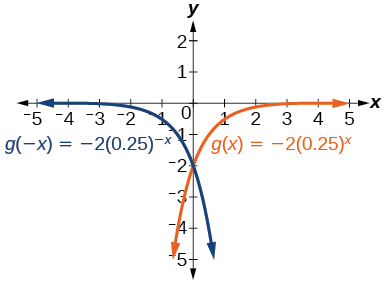y -intercept: $\text{\hspace{0.17em}}\left(0,-2\right)$

$h\left(x\right)=6{\left(1.75\right)}^{-x}$

For the following exercises, graph each set of functions on the same axes.

$f\left(x\right)=3{\left(\frac{1}{4}\right)}^{x},$ $g\left(x\right)=3{\left(2\right)}^{x},$ and $\text{\hspace{0.17em}}h\left(x\right)=3{\left(4\right)}^{x}$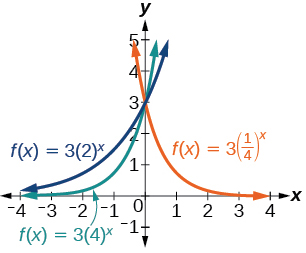$f\left(x\right)=\frac{1}{4}{\left(3\right)}^{x},$ $g\left(x\right)=2{\left(3\right)}^{x},$ and $\text{\hspace{0.17em}}h\left(x\right)=4{\left(3\right)}^{x}$

For the following exercises, match each function with one of the graphs in [link] .

$f\left(x\right)=2{\left(0.69\right)}^{x}$

B

$f\left(x\right)=2{\left(1.28\right)}^{x}$

$f\left(x\right)=2{\left(0.81\right)}^{x}$

A

$f\left(x\right)=4{\left(1.28\right)}^{x}$

$f\left(x\right)=2{\left(1.59\right)}^{x}$

E

$f\left(x\right)=4{\left(0.69\right)}^{x}$

For the following exercises, use the graphs shown in [link] . All have the form $\text{\hspace{0.17em}}f\left(x\right)=a{b}^{x}.$

Which graph has the largest value for $\text{\hspace{0.17em}}b?$

D

Which graph has the smallest value for $\text{\hspace{0.17em}}b?$

Which graph has the largest value for $\text{\hspace{0.17em}}a?$

C

Which graph has the smallest value for $\text{\hspace{0.17em}}a?$

For the following exercises, graph the function and its reflection about the x -axis on the same axes.

$f\left(x\right)=\frac{1}{2}{\left(4\right)}^{x}$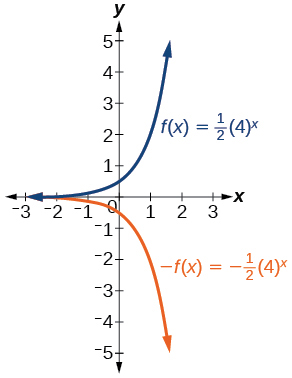$f\left(x\right)=3{\left(0.75\right)}^{x}-1$

$f\left(x\right)=-4{\left(2\right)}^{x}+2$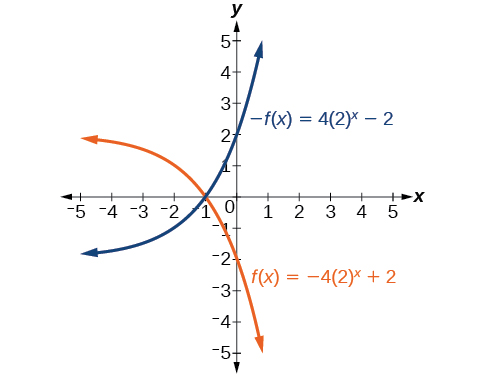For the following exercises, graph the transformation of $\text{\hspace{0.17em}}f\left(x\right)={2}^{x}.\text{\hspace{0.17em}}$ Give the horizontal asymptote, the domain, and the range.

$f\left(x\right)={2}^{-x}$

$h\left(x\right)={2}^{x}+3$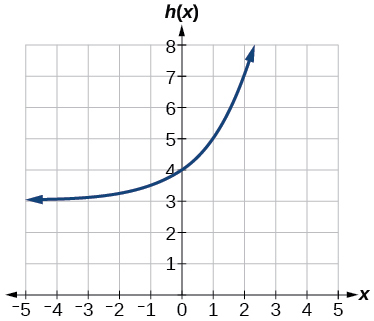Horizontal asymptote: $\text{\hspace{0.17em}}h\left(x\right)=3;$ Domain: all real numbers; Range: all real numbers strictly greater than $\text{\hspace{0.17em}}3.$

$f\left(x\right)={2}^{x-2}$

For the following exercises, describe the end behavior of the graphs of the functions.

$f\left(x\right)=-5{\left(4\right)}^{x}-1$

As $x\to \infty$ , $f\left(x\right)\to -\infty$ ;
As $x\to -\infty$ , $f\left(x\right)\to -1$

$f\left(x\right)=3{\left(\frac{1}{2}\right)}^{x}-2$

$f\left(x\right)=3{\left(4\right)}^{-x}+2$

As $x\to \infty$ , $f\left(x\right)\to 2$ ;
As $x\to -\infty$ , $f\left(x\right)\to \infty$

For the following exercises, start with the graph of $\text{\hspace{0.17em}}f\left(x\right)={4}^{x}.\text{\hspace{0.17em}}$ Then write a function that results from the given transformation.

Shift $f\left(x\right)$ 4 units upward

Shift $\text{\hspace{0.17em}}f\left(x\right)\text{\hspace{0.17em}}$ 3 units downward

$f\left(x\right)={4}^{x}-3$

Shift $\text{\hspace{0.17em}}f\left(x\right)\text{\hspace{0.17em}}$ 2 units left

Shift $\text{\hspace{0.17em}}f\left(x\right)\text{\hspace{0.17em}}$ 5 units right

$f\left(x\right)={4}^{x-5}$

Reflect $\text{\hspace{0.17em}}f\left(x\right)\text{\hspace{0.17em}}$ about the x -axis

Reflect $\text{\hspace{0.17em}}f\left(x\right)\text{\hspace{0.17em}}$ about the y -axis

$f\left(x\right)={4}^{-x}$

For the following exercises, each graph is a transformation of $\text{\hspace{0.17em}}y={2}^{x}.\text{\hspace{0.17em}}$ Write an equation describing the transformation.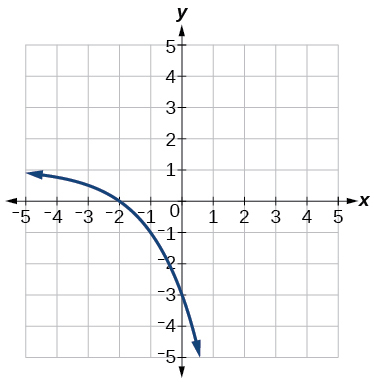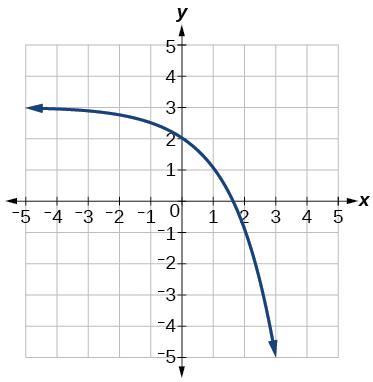$y=-{2}^{x}+3$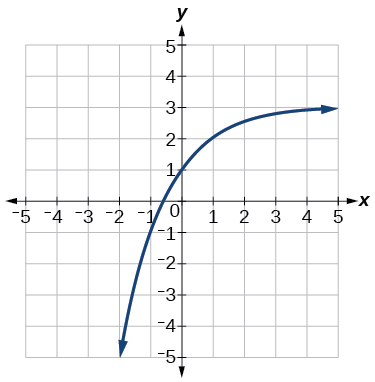For the following exercises, find an exponential equation for the graph.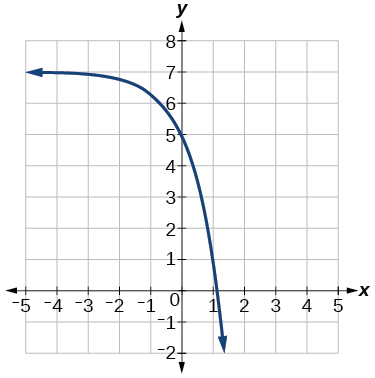$y=-2{\left(3\right)}^{x}+7$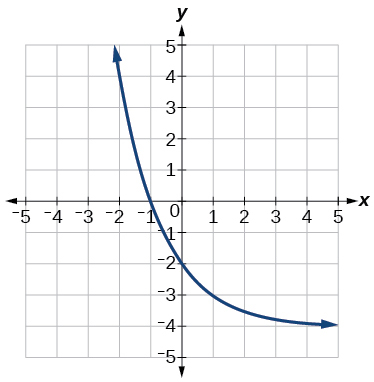## Numeric

For the following exercises, evaluate the exponential functions for the indicated value of $\text{\hspace{0.17em}}x.$

$g\left(x\right)=\frac{1}{3}{\left(7\right)}^{x-2}\text{\hspace{0.17em}}$ for $\text{\hspace{0.17em}}g\left(6\right).$

$g\left(6\right)=800+\frac{1}{3}\approx 800.3333$

$f\left(x\right)=4{\left(2\right)}^{x-1}-2\text{\hspace{0.17em}}$ for $\text{\hspace{0.17em}}f\left(5\right).$

$h\left(x\right)=-\frac{1}{2}{\left(\frac{1}{2}\right)}^{x}+6\text{\hspace{0.17em}}$ for $\text{\hspace{0.17em}}h\left(-7\right).$

$h\left(-7\right)=-58$

## Technology

For the following exercises, use a graphing calculator to approximate the solutions of the equation. Round to the nearest thousandth. $\text{\hspace{0.17em}}f\left(x\right)=a{b}^{x}+d.$

$-50=-{\left(\frac{1}{2}\right)}^{-x}$

$116=\frac{1}{4}{\left(\frac{1}{8}\right)}^{x}$

$x\approx -2.953$

$12=2{\left(3\right)}^{x}+1$

$5=3{\left(\frac{1}{2}\right)}^{x-1}-2$

$x\approx -0.222$

$-30=-4{\left(2\right)}^{x+2}+2$

## Extensions

Explore and discuss the graphs of $\text{\hspace{0.17em}}F\left(x\right)={\left(b\right)}^{x}\text{\hspace{0.17em}}$ and $\text{\hspace{0.17em}}G\left(x\right)={\left(\frac{1}{b}\right)}^{x}.\text{\hspace{0.17em}}$ Then make a conjecture about the relationship between the graphs of the functions $\text{\hspace{0.17em}}{b}^{x}\text{\hspace{0.17em}}$ and $\text{\hspace{0.17em}}{\left(\frac{1}{b}\right)}^{x}\text{\hspace{0.17em}}$ for any real number $\text{\hspace{0.17em}}b>0.$

The graph of $\text{\hspace{0.17em}}G\left(x\right)={\left(\frac{1}{b}\right)}^{x}\text{\hspace{0.17em}}$ is the refelction about the y -axis of the graph of $\text{\hspace{0.17em}}F\left(x\right)={b}^{x};\text{\hspace{0.17em}}$ For any real number $\text{\hspace{0.17em}}b>0\text{\hspace{0.17em}}$ and function $\text{\hspace{0.17em}}f\left(x\right)={b}^{x},$ the graph of $\text{\hspace{0.17em}}{\left(\frac{1}{b}\right)}^{x}\text{\hspace{0.17em}}$ is the the reflection about the y -axis, $\text{\hspace{0.17em}}F\left(-x\right).$

Prove the conjecture made in the previous exercise.

Explore and discuss the graphs of $\text{\hspace{0.17em}}f\left(x\right)={4}^{x},$ $\text{\hspace{0.17em}}g\left(x\right)={4}^{x-2},$ and $\text{\hspace{0.17em}}h\left(x\right)=\left(\frac{1}{16}\right){4}^{x}.\text{\hspace{0.17em}}$ Then make a conjecture about the relationship between the graphs of the functions $\text{\hspace{0.17em}}{b}^{x}\text{\hspace{0.17em}}$ and $\text{\hspace{0.17em}}\left(\frac{1}{{b}^{n}}\right){b}^{x}\text{\hspace{0.17em}}$ for any real number n and real number $\text{\hspace{0.17em}}b>0.$

The graphs of $\text{\hspace{0.17em}}g\left(x\right)\text{\hspace{0.17em}}$ and $\text{\hspace{0.17em}}h\left(x\right)\text{\hspace{0.17em}}$ are the same and are a horizontal shift to the right of the graph of $\text{\hspace{0.17em}}f\left(x\right);\text{\hspace{0.17em}}$ For any real number n , real number $\text{\hspace{0.17em}}b>0,$ and function $\text{\hspace{0.17em}}f\left(x\right)={b}^{x},$ the graph of $\text{\hspace{0.17em}}\left(\frac{1}{{b}^{n}}\right){b}^{x}\text{\hspace{0.17em}}$ is the horizontal shift $\text{\hspace{0.17em}}f\left(x-n\right).$

Prove the conjecture made in the previous exercise.

how fast can i understand functions without much difficulty
what is set?
a colony of bacteria is growing exponentially doubling in size every 100 minutes. how much minutes will it take for the colony of bacteria to triple in size
I got 300 minutes. is it right?
Patience
no. should be about 150 minutes.
Jason
It should be 158.5 minutes.
Mr
ok, thanks
Patience
100•3=300 300=50•2^x 6=2^x x=log_2(6) =2.5849625 so, 300=50•2^2.5849625 and, so, the # of bacteria will double every (100•2.5849625) = 258.49625 minutes
Thomas
what is the importance knowing the graph of circular functions?
can get some help basic precalculus
What do you need help with?
Andrew
how to convert general to standard form with not perfect trinomial
can get some help inverse function
ismail
Rectangle coordinate
how to find for x
it depends on the equation
Robert
yeah, it does. why do we attempt to gain all of them one side or the other?
Melissa
whats a domain
The domain of a function is the set of all input on which the function is defined. For example all real numbers are the Domain of any Polynomial function.
Spiro
Spiro; thanks for putting it out there like that, 😁
Melissa
foci (–7,–17) and (–7,17), the absolute value of the differenceof the distances of any point from the foci is 24.
difference between calculus and pre calculus?
give me an example of a problem so that I can practice answering
x³+y³+z³=42
Robert
dont forget the cube in each variable ;)
Robert
of she solves that, well ... then she has a lot of computational force under her command ....
Walter
what is a function?
I want to learn about the law of exponent
explain this

#### Get Jobilize Job Search Mobile App in your pocket Now!ByByBy Melinda SalzerBy OpenStaxBy Kimberly NicholsBy Caitlyn GobbleBy Eddie UnverzagtBy OpenStaxBy RhodesBy Brooke DelaneyBy OpenStaxBy OpenStax